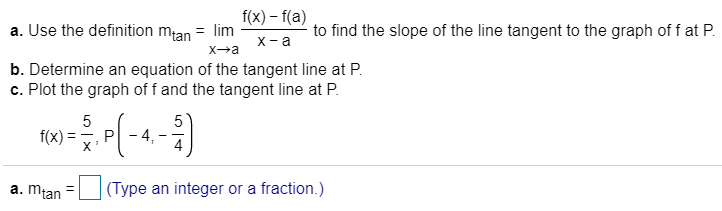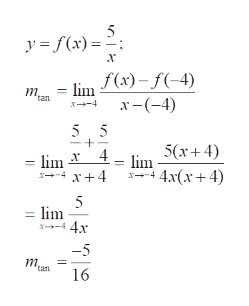# a. Use the definition mtan = lim I(x) - f(a)to find the slope of the line tangent to the graph of f at PX aXab. Determine an equation of the tangent line at Pc. Plot the graph of f and the tangent line at P554f(x)PX(Type an integer or a fraction.)a. mtan

Question
92 viewshelp_outlineImage Transcriptionclosea. Use the definition mtan = lim I(x) - f(a) to find the slope of the line tangent to the graph of f at P X a Xa b. Determine an equation of the tangent line at P c. Plot the graph of f and the tangent line at P 5 5 4 f(x) P X (Type an integer or a fraction.) a. mtan fullscreen
check_circle

Step 1

(a) to determine the slope of the tangent at the point P to the given curve y=f(x), using the limit definition of the derivativehelp_outlineImage Transcriptionclosey =f(x) х - lim f(x) - f(-4) x-(-4) т. tan x4 5 5 5(x4) 4 lim 4 4 4 4x(x+ 4) - lim lim x-44 -5 m. "tan 16 + fullscreen
Step 2

Equation of the tangent at the ...

### Want to see the full answer?

See Solution

#### Want to see this answer and more?

Solutions are written by subject experts who are available 24/7. Questions are typically answered within 1 hour.*

See Solution
*Response times may vary by subject and question.
Tagged in

### Calculus